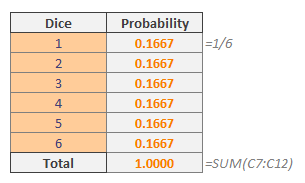A discrete distribution describes the probability of occurrence of a random variable that can take on only a certain number of values. Common examples for this are the probabilities in a dice roll or getting a certain card in a deck of regular cards. In this guide, we’re going to show you how to calculate discrete probability in Excel.

## What is probability?

Probability is a branch of mathematics that shows how likely an event is to occur. In a nutshell, it is the ratio of the number of occurrences of an event to the total number of occurrences.

Let’s look at rolling a dice. A standard dice has 6 sides and  each side has an equal chance to be on top. Thus, we can say each number has 1/6 = 0.1667 probability.If you want to calculate the probability of getting 1 or 2 or 3 on a dice roll, you can sum up the probability values or use the PROB function.

## PROB function

The PROB function is a statistical function that can calculate the probability associated with a given range. The function allows you to set lower and upper limits to be able to calculate probability between.

PROB(x_range, prob_range, [lower_limit], [upper_limit])

 x_range The range of numeric values which have associated probabilities. prob_range The range of probabilities associated with values in x_range. lower_limit The lower bound on the value for which you want to calculate the probability. [upper_limit] Optional. The lower bound on the value for which you want to calculate the probability.If this is omitted, the PROB function returns the probability for the x_range values equal to the lower_limit.

## How to calculate discrete probability with PROB function

The first argument of the PROB function, x_range, accepts events by numerical values. Events, in this example, are the numbers of a dice. The second argument, prob_range, is for the probabilities of occurrences of the corresponding events. The rest of the arguments are for the lower and upper limits, respectively.

To return the probability of getting 1 or 2 or 3 on a dice roll, the data and formula should be like the following:=PROB(B7:B12,C7:C12,1,3)

The formula returns 0.5, which means you have a 50% chance to get 1 or 2 or 3 from a single roll. Let’s check a more complex example for calculating discrete probability with 2 dices.

When you roll two dices, you can get numbers between 2 and 12. However, the probabilities of numbers are not equal this time. The table of numbers and probability values are below.If your aim is to find the probability of a single event, you can use the COUNTIF function to count values above, based on the event value and divide it by the total number of events. You can find total number by multiplying dice numbers (6 * 6) or counting them using the COUNT function.

You can see that the most likely result is the number 7 with a probability of 0.1667.